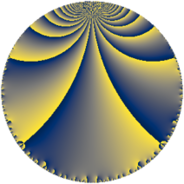# Properties

 Label 147.6.oLevel $147$ Weight $6$ Character orbit 147.o Rep. character $\chi_{147}(5,\cdot)$ Character field $\Q(\zeta_{42})$ Dimension $1092$ Sturm bound $112$

# Related objects

## Defining parameters

 Level: $$N$$ $$=$$ $$147 = 3 \cdot 7^{2}$$ Weight: $$k$$ $$=$$ $$6$$ Character orbit: $$[\chi]$$ $$=$$ 147.o (of order $$42$$ and degree $$12$$) Character conductor: $$\operatorname{cond}(\chi)$$ $$=$$ $$147$$ Character field: $$\Q(\zeta_{42})$$ Sturm bound: $$112$$

## Dimensions

The following table gives the dimensions of various subspaces of $$M_{6}(147, [\chi])$$.

Total New Old
Modular forms 1140 1140 0
Cusp forms 1092 1092 0
Eisenstein series 48 48 0

## Trace form

 $$1092 q - 11 q^{3} - 1434 q^{4} + 266 q^{6} - 113 q^{7} + 1507 q^{9} + O(q^{10})$$ $$1092 q - 11 q^{3} - 1434 q^{4} + 266 q^{6} - 113 q^{7} + 1507 q^{9} + 722 q^{10} + 2265 q^{12} - 28 q^{13} + 590 q^{15} + 20902 q^{16} + 2559 q^{18} - 1137 q^{19} + 4486 q^{21} - 8480 q^{22} - 9572 q^{24} + 48553 q^{25} - 14105 q^{27} - 988 q^{28} + 9077 q^{30} + 21867 q^{31} - 28688 q^{33} - 43092 q^{34} - 24038 q^{36} + 55738 q^{37} + 10053 q^{39} - 210318 q^{40} - 4312 q^{42} - 57946 q^{43} - 56927 q^{45} + 239492 q^{46} + 114071 q^{49} - 24596 q^{51} + 13412 q^{52} - 114548 q^{54} - 64498 q^{55} + 130814 q^{57} - 91938 q^{58} + 52620 q^{60} - 89267 q^{61} + 74395 q^{63} + 478240 q^{64} + 279528 q^{66} + 20105 q^{67} + 331702 q^{69} - 848652 q^{70} - 185082 q^{72} - 376783 q^{73} + 30475 q^{75} - 132132 q^{76} + 22488 q^{78} + 234973 q^{79} + 1133087 q^{81} + 100030 q^{82} - 197548 q^{84} - 13156 q^{85} - 338590 q^{87} - 88430 q^{88} - 937475 q^{90} + 307711 q^{91} - 40325 q^{93} - 293516 q^{94} + 560728 q^{96} - 553432 q^{99} + O(q^{100})$$

## Decomposition of $$S_{6}^{\mathrm{new}}(147, [\chi])$$ into newform subspaces

The newforms in this space have not yet been added to the LMFDB.